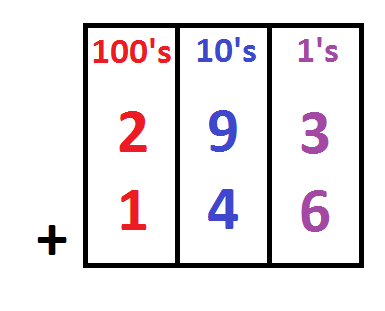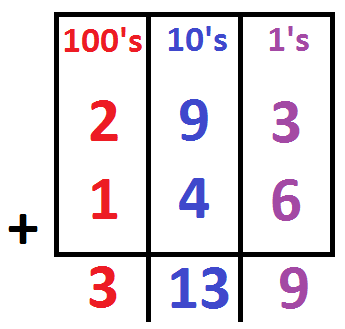Instructor: Allison Petrovic

Allison has experience teaching high school and college mathematics and has a master's degree in mathematics education.

In this lesson, we will learn how to add large numbers using a fun, simple method called the column addition method. We will first go over place values, and then we will learn this three-step method!

## Collecting Pennies

Suppose that you enjoy collecting pennies. You found 239 pennies last month and this month you have collected 146 pennies so far. You are excited to figure out how many pennies you have collected in total. We can use the column addition method to help us easily calculate how many total pennies you have.

## Place Value

It is important that we understand the place values of each individual digit before we use the column addition method to help us count our pennies. Let's review the place values that we will be using in this lesson.

Suppose that we have the number 123. In this number we have three digits. We have the digits 1, 2, and 3! The digit 3 is in the ones place. The digit 2 is in the tens place. The digit 1 is in the hundreds place. Think about if you were to say the number 123. You would say out loud 'one hundred twenty-three'. This also confirms that the 1 digit is in the hundreds place (100), the 2 digit is in the tens place (20), and the 3 is in the ones place (3). Now, let's learn the three simple steps to the column addition method.

### Step 1

#### Create columns for each place value

When adding 239 and 146, we can see that both numbers have three digits. So we will need to create three columns for the column addition method. We will create a hundreds column, tens column, and ones column.### Step 2

#### Add the digits in each column

We will add 3 + 6 = 9 for the ones column. 9 + 4 = 13 for the tens column. 2 + 1 = 3 for the hundreds column.To unlock this lesson you must be a Study.com Member.

### Register to view this lesson

Are you a student or a teacher?

#### See for yourself why 30 million people use Study.com

##### Become a Study.com member and start learning now.
Back
What teachers are saying about Study.com

### Earning College Credit

Did you know… We have over 200 college courses that prepare you to earn credit by exam that is accepted by over 1,500 colleges and universities. You can test out of the first two years of college and save thousands off your degree. Anyone can earn credit-by-exam regardless of age or education level.